# power

(redirected from Power (disambiguation))
Also found in: Dictionary, Thesaurus, Legal, Encyclopedia.

## power

1. capability; the ability to act.
2. the ability of a statistical test to detect statistically significant differences when they exist.
durable power of attorney for health care advance directives.
Miller-Keane Encyclopedia and Dictionary of Medicine, Nursing, and Allied Health, Seventh Edition. © 2003 by Saunders, an imprint of Elsevier, Inc. All rights reserved.

## pow·er

(pow'ĕr),
1. In optics, the refractive vergence of a lens.
2. In physics and engineering, the rate at which work is done.
3. The exponent of a number or expression that provides the number of times that number has to be multiplied by itself.
Farlex Partner Medical Dictionary © Farlex 2012

## power

Clinical research The probability of detecting a treatment effect of a given magnitude when a treatment effect of at least that magnitude truly exists Vox populi Energy. See Crypto-nuclear power, Durable power of attorney, Guild power, Intellectual processing power, Mass stopping power, Null hypothesis, Police power, Pyramid power, Scanning power, Social power.
McGraw-Hill Concise Dictionary of Modern Medicine. © 2002 by The McGraw-Hill Companies, Inc.

## pow·er

(pow'ĕr)
1. optics The refractive vergence of a lens.
2. physics The rate at which work is done.
3. The product of force and velocity (distance divided by time) expressed in watts.
Medical Dictionary for the Health Professions and Nursing © Farlex 2012

## power

General term that may refer to any power such as effective, equivalent, dioptric, focal, refractive, surface or vergence power.
aligning power  See vernier visual acuity.
approximate power See nominal power.
back vertex power (BVP) The reciprocal of the back vertex focal length. It is equal to
Fv = n′/SF
where n′ is the refractive index of the second medium, S is the point on the back surface through which passes the optical axis and F′ the second principal focus. Symbol: Fv. Other formulae for the back vertex power of a lens (or an optical system) are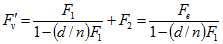where d is the thickness of the lens, n the index of refraction of the lens, F1 the power of the front surface, F2 the power of the back surface and Fe the equivalent power. The powers are in dioptres and the length in metres. The back vertex power is the usual measurement made by a focimeter. Syn. back power. See vergence; vertex focal length.
dioptric power See refractive power.
dispersive power See dispersion.
effective power The power of a lens or surface measured in a plane other than the principal plane and usually remote from the lens or surface. If a thin lens or surface of power F is illuminated by parallel incident light, the effective power Fx of another lens placed at a distance d from the original lens and forming an image in the same position, is given by the equationwhere d is in metres and positive when measured from left to right. Examples: (1) If a hyperopic eye is corrected by a lens F = +5 D placed 12 mm from the cornea, the ocular refraction is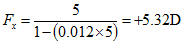(2) If an eye has an ocular refraction of −10D, its spectacle refraction at a vertex distance of 10 mm is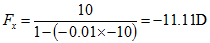See vertex distance.
equivalent power The refractive power of a lens or an optical system expressed with reference to the principal points. It corresponds to the refractive power of a thin lens placed in the second principal plane which would form an image of a distant object of the same size as that produced by the system that it replaces. It is equal to
Fe = n′/f′ = − n/f
where n and n′ are the indices of refraction of the object and image space, respectively, f and f′ the distances (in metres) between the first and second principal points and the first and second principal foci, respectively. The equivalent power (symbol: Fe) is in dioptres. It is also equal to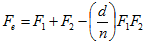where F1 and F2 are the powers of the lenses or surfaces comprising the system, d is the distance between the two and n the index of refraction of the intervening medium. Example: If the anterior surface power of the cornea is equal to +48.21 D, the posterior surface power is equal to −5.97 D, the thickness of the cornea 0.5 mm and the index of refraction 1.376, the equivalent power will be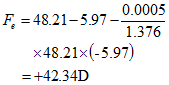Syn. true power. See equivalent focal length; nominal power.
equivalent viewing power (EVP) A term used to describe the magnifying effect of a lens (or lens system). It is equal to the power resulting from the combination of a magnifier Fm assumed to be a thin lens and a near addition (or the accommodation exerted) Fa, i.e. EVP = Fm + Fa − zFm Fa where z is the distance (in metres) between the magnifier and the eye (or spectacle plane). EVP may also be expressed as EVP = MFa, where M is the enlargement ratio (lateral magnification). If the magnifier is placed against the spectacle lens (z = 0), EVP = Fm + Fa. If the magnifier is placed against the object being viewed (z = fa), EVP = Fa. If the magnifier is held at its focal length from the eye (z = fm), EVP = Fm: EVP is then equal to the power of the magnifier, irrespective of any near addition (or accommodation). Example: A patient uses a lens +16 D placed 12 cm from the eye and wears a near addition of +4.00 D. EVP = 16 + 4 − 0.12 ✕ 16 ✕ 4 = +12.3 D, the enlargement ratio (M = EVP/Fa) is 12.3 / 4 = 3.1✕, and at a distance of 4 cm from the eye the enlargement is equal to 4.4✕, whereas the conventional magnification remains Fm/4 = 4✕.The reciprocal of EVP is called the equivalent viewing distance (EVD). It represents the focal length of the equivalent magnifying system where the target must be placed to be seen clearly. See lateral magnification.
power factor See spectacle magnification.
focal power See fundamental paraxial equation; refractive power.
front vertex power (FVP) The reciprocal of the front vertex focal length. It is equal to
Fv = n/SF
where n is the refractive index of the first medium, S is the point on the front surface through which passes the optical axis and F is the first principal focus. Symbol: Fv. Other formulae for the front vertex power of a lens (or an optical system) are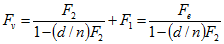where d is the thickness of the lens, n the index of refraction of the lens, F1 the power of the front surface, F2 the power of the back surface and Fe the equivalent power. The powers are in dioptres and the length in metres. Syn. front power. See effective power; equivalent power; vergence; vertex focal length.
magnification power See spectacle magnification.
magnifying power See apparent magnification.
nominal power An estimate of the power of a lens, calculated as the sum of the front and back surface powers, i.e.
F = F1 + F2
Syn. approximate power. See equivalent power; surface power.
prism power The amount of deviation of a ray of light transmitted through a prism or lens (outside its optical centre). It is usually expressed in prism dioptres (D) and given by the following approximate formula for small angle prisms (in air)
P = 100(n − 1)a
where a is the prism angle in radians and n the index of refraction of the prism. Example: What is the power of a prism with an apex angle of 6º and a refractive index of 1.50? P = 100(1.50 − 1) (6/57.3) = 5.24 Χ which corresponds to the deviation of a ray of light equal to 5.24 cm at 100 cm. Syn. prismatic power. See prism dioptre; Prentice's law.
prismatic power See prism power.
refractive power The ability of a lens or an optical system to change the direction of a pencil of rays. It is equal to
F = n′/f′ = −n/f
where n and n′ are the refractive indices of the object and image space, respectively, f and f′ the first and second focal length, respectively, in metres, and the power F is expressed in dioptres. Symbol: F. Syn. dioptric power; focal power; vergence power. See fundamental paraxial equation; equivalent power; vergence.
resolving power See limit of resolution.
surface power The dioptric power of a single refracting or reflecting surface. It is equal to
F = n′ − n/r
where F is the power in dioptres, n and n′ are the refractive indices of the media on each side of the surface and r is the radius of curvature of the lens or mirror surface, in metres. This equation forms part of the fundamental paraxial equation. For a spectacle lens in air (n = 1) the power of the surface becomes
F = n′ − 1/r
Examples: Power of the corneal surfaces.(1) Anterior surface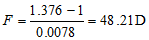where the refractive index of the cornea is 1.376 and the surface has a radius of curvature of 7.8 mm.(2) Posterior surface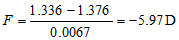where the refractive indices of the aqueous humour and the cornea are 1.336 and 1.376, respectively, and the surface has a radius of curvature of 6.7 mm.For a thin spectacle lens in air, the sum of the powers of the two surfaces F1 + F2 represents the total power of the lens and is equal towhere n is the index of refraction of the lens and r1 and r2 the radii of curvature of its two surfaces. See fundamental paraxial equation.
true power See equivalent power.
vergence power See fundamental paraxial equation; refractive power; vergence.
vertex power See back vertex power; front vertex power.
 Table P5 Power (in dioptres) of the surfaces and structures of an average adult Caucasian eye* anterior surface of the cornea 48.21 posterior surface of the cornea −5.97 complete corneal system 42.34 anterior surface of the lens 7.92 accommodated 13.77 posterior surface of the lens 13.54 accommodated 15.84 complete lens system 21.19 accommodated 29.42 complete eye 59.44 accommodated 67.56 refraction of the eye +0.50 ocular accommodation 8.12 *see constants of the eye.

 Table P6 Powers of the surfaces of contact lenses of thickness d = 0.20 mm, in which the radius of curvature of the back optic zone is constant and that of the front optic zone varies to produce various back vertex powers and equivalent powers. The index of refraction of these lenses is assumed to be 1.49 radius of back optic zone (mm) back surface power (D) F2 radius of front optic zone (mm) front surface power (D) F1 power of lens (D) considered thin F1 1 F2 equivalent power (D) Fe back vertex power (D) F′v 7.8 −62.82 7.2 68.06 +5.24 +5.81 +5.86 7.8 −62.82 7.4 66.22 +3.40 +3.95 +3.99 7.8 −62.82 7.6 64.47 +1.65 +2.20 +2.22 7.8 −62.82 7.8 62.82 0.00 +0.53 +0.53 7.8 −62.82 8.0 61.25 −1.57 −1.05 −1.06 7.8 −62.82 8.2 59.76 −3.06 −2.56 −2.58 7.8 −62.82 8.4 58.33 −4.49 −4.00 −4.03 7.8 −62.82 8.6 56.98 −5.84 −5.36 −5.40 7.8 −62.82 8.8 55.68 −7.14 −6.67 −6.72 7.8 −62.82 9.0 54.44 −8.38 −7.92 −7.98 7.8 −62.82 9.2 53.26 −9.56 −9.11 −9.18 7.8 −62.82 9.4 52.13 −10.69 −10.25 −10.33

 Table P7 Contact lens power (or ocular refraction) corresponding to a spectacle lens situated at two vertex distances spectacle lens power (D) contact lens power (D) 10 mm 14 mm −16 −13.79 −13.07 −14 −12.28 −11.71 −12 −10.71 −10.27 −10 −9.09 −8.77 −9 −8.26 −7.99 −8 −7.41 −7.19 −7 −6.54 −6.38 −6 −5.66 −5.53 −5 −4.76 −4.67 −4 −3.85 −3.79 −3 −2.91 −2.88 +3 +3.09 +3.13 +4 +4.17 +4.24 +5 +5.26 +5.38 +6 +6.38 +6.55 +7 +7.53 +7.76 +8 +8.70 +9.01 +9 +9.89 +10.30 +10 +11.11 +11.63 +12 +13.64 +14.42

 Table P8 Surface power of the anterior surface of the cornea (in dioptres) corresponding to various radii of curvature (in mm). Calculations were made using 1.376 as the index of refraction of the cornea radius power radius power 6.80 55.29 7.80 48.20 7.00 53.71 7.85 47.90 7.10 52.96 7.90 47.59 7.20 52.22 7.95 47.30 7.30 51.51 8.00 47.00 7.40 50.81 8.10 46.42 7.50 50.13 8.20 45.85 7.55 49.80 8.30 45.30 7.60 49.47 8.40 44.76 7.65 49.15 8.50 44.23 7.70 48.83 8.60 43.72 7.75 48.52 8.80 42.73
Millodot: Dictionary of Optometry and Visual Science, 7th edition. © 2009 Butterworth-Heinemann

## Patient discussion about power

Q. My myopic son is wearing power glasses. Are there any other nutritional supplements to support eye sight? My myopic son is wearing power glasses from the age of 2 years. His power is not very high yet but the rate of his eye power is doubling every year. Doctor had given him some medicines and had told him to have lots of carrots. We are giving him carrot juice every day. But soon he stopped taking it for some months. But he is having juice now but I wish to know are there any other nutritional supplements to support eye sight?

A. eating carrots can help people who suffers from vitamin A or beta-carotene deficiency. which leads to poor night vision. but that's it. there is no reason to eat tremendous amounts of carrots, there are food supplements that will help you achieve it without becoming orange. anyway, getting too much vitamin A can be toxic.
here is a "snopes" about it-
http://www.snopes.com/food/ingredient/carrots.asp

More discussions about power
This content is provided by iMedix and is subject to iMedix Terms. The Questions and Answers are not endorsed or recommended and are made available by patients, not doctors.
Site: Follow: Share:
Open / Close